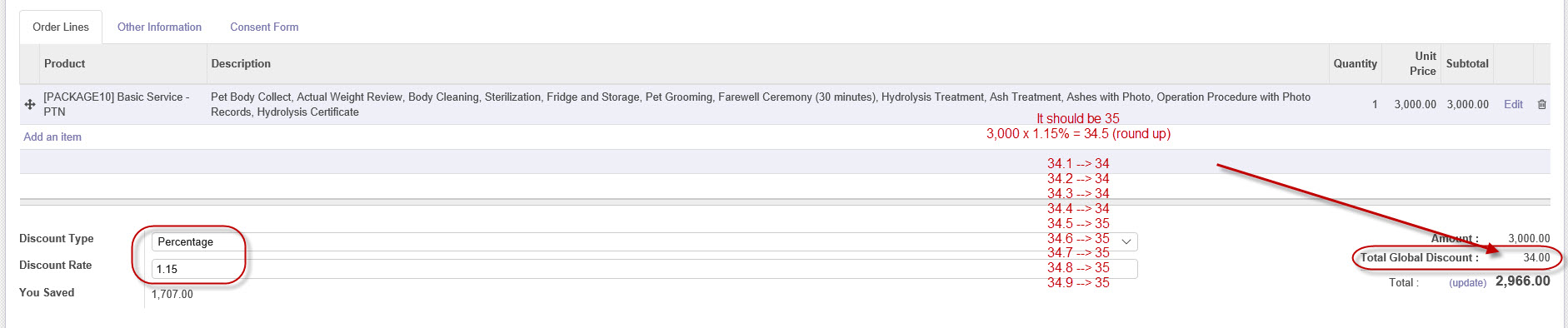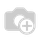# Using round function still price 34.50 round to 34 in Odoo10

Hello All,

I'm using round value for floated price but still my price 34.50 is rounded to 34. It should be 35 when it reached to 34.50. Mainly its work fine but i don't understand why it is not working on 3000 price percentage discount is 1.15

I am also attaching screen and code also.

`@api.one@api.depends('discount_type','discount_rate','amount_total')def _compute_discount(self):    mod_obj = self.env['ir.model.data']    amount_discount = 0.0    if self.discount_type == 'percent':        amount_discount = self.amount_untaxed * self.discount_rate / 100    else:        amount_discount = self.discount_rate    self.amount_discount = round(amount_discount)`Hi Pawan,

This is due to an issue with floating points in python and in fact most programming languages.

Putting 3000 * 1.15 / 100.0 into a python terminal will actually give you 34.49999999999999, which is then rounded down to 34.

As they are prices, you could use round(round(amount_discount, 2)) to round it to 34.50 and then to 35. In general it means anything > .495 is rounded up.

Regards,

Jake Robinson

1 CommentHi Pawan Sharrma,